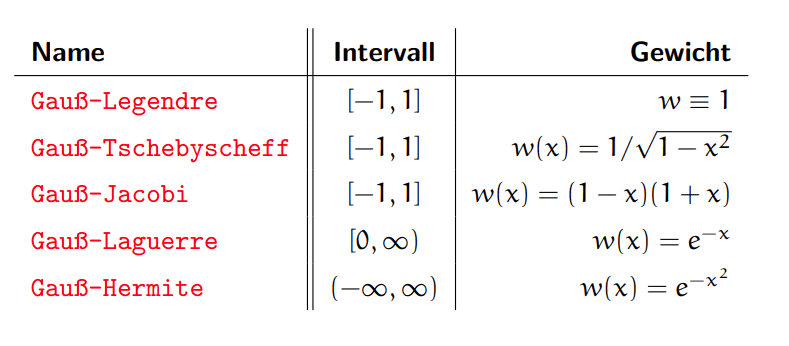• MHB
Gold Member
MHB
Hey! 😊

If we want to calculate the nodes $x_1, x_2$ and the weight functions $w_1, w_2$ for the Gaussian quadrature of the integral $$\int_{-1}^1f(x)\, dx\approx \sum_{j=1}^2w_jf(x_j)$$ is there a criteria that we have to consider at chosing the weight functions? I mean if we use e.g. Gauss-Legendre or Tschebyscheff-Jacobi? :unsure:

Homework Helper
MHB
Hi mathmari!

We can choose the weights in multiple ways. It depends on the function $f$ what the best one is.
Gauss-Legendre is for an $f$ that is "well approximated by polynomials on [-1,1]", which will not always be the case.
I cannot find Tschebyscheff-Jacobi, but Chebyshev–Gauss is for a specific form of $f$. 🤔

Gold Member
MHB
We can choose the weights in multiple ways. It depends on the function $f$ what the best one is.
Gauss-Legendre is for an $f$ that is "well approximated by polynomials on [-1,1]", which will not always be the case.
I cannot find Tschebyscheff-Jacobi, but Chebyshev–Gauss is for a specific form of $f$. 🤔

In this exercise we have the function $e^{-\frac{1}{x^2}-x^2}$.

Which method is the best here? :unsure:

Homework Helper
MHB
In this exercise we have the function $e^{-\frac{1}{x^2}-x^2}$.

Which method is the best here?

I don't know which Gaussian Quadrature would be best for that.
If it were me, I'd use Simpson's rule instead, which is simpler and which will work. 🤔

Gold Member
MHB
I don't know which Gaussian Quadrature would be best for that.
If it were me, I'd use Simpson's rule instead, which is simpler and which will work. 🤔

So in general can we use one of the Gaussian Quadrature methods? Or is there a specific criteria? :unsure:

Gold Member
MHB
According to the exercise statement we have to calculate the nodes and the weight functions. So do we have to calculate them by ourselves instead of taking the already known functions Gauss-Legendre, Chebyshev, etc? :unsure:

Homework Helper
MHB
Since the function does not match any of the special functions in the wiki article, I think we should use the generic Gauss–Legendre version.
Then we can still calculate the weights based on the number of points that we choose. 🤔

Gold Member
MHB
Since the function does not match any of the special functions in the wiki article, I think we should use the generic Gauss–Legendre version.
Then we can still calculate the weights based on the number of points that we choose. 🤔

I found now the below table:Does this mean that since we have an integral of the formm $\int_{-1}^1f(x)\, dx$, i.e. $w\equiv 1$ we use the Gauss-Legendre?
If we would have an integral of the form $\int_{-1}^1f(x)\cdot \frac{1}{\sqrt{1-x^2}}\, dx$ we would use Gauss-Tschebyscheff, etc?

Or since we have the function $f(x)=e^{-\frac{1}{x^2}-x^2}$ we write it in the form $e^{-\frac{1}{x^2}}e^{-x^2}$ and we use Gauss-Laguerre for example consider the function $e^{-\frac{1}{x^2}}$ ?

:unsure:

Homework Helper
MHB
Does this mean that since we have an integral of the formm $\int_{-1}^1f(x)\, dx$, i.e. $w\equiv 1$ we use the Gauss-Legendre?
If we would have an integral of the form $\int_{-1}^1f(x)\cdot \frac{1}{\sqrt{1-x^2}}\, dx$ we would use Gauss-Tschebyscheff, etc?

It depends on what $f(x)$ is. If it is a function that behaves like a polynomial, you are right.
If $f(x)$ is a polynomial divided by $\sqrt{1-x^2}$, we should use Gauss-Tschebyscheff. (Wondering)

Or since we have the function $f(x)=e^{-\frac{1}{x^2}-x^2}$ we write it in the form $e^{-\frac{1}{x^2}}e^{-x^2}$ and we use Gauss-Laguerre for example consider the function $e^{-\frac{1}{x^2}}$ ?
That's a possibility yes. 🤔

Gold Member
MHB
What do you suggest me to do in this exercise, becaus I am confused right now? To use ready functions or to calculate by myself some weight functions? :unsure:

Gold Member
MHB
Do we suppose that the formula has to be exact for polynomials till degree $n=3$ and so we get:
Sei $f(x)=1$ dann $$\int_{-1}^11\, dx=2\approx w_1+w_2 \\ \int_{-1}^1x\, dx=0\approx w_1x_1+w_2x_2 \\ \int_{-1}^1x^2\, dx=\frac{2}{3}\approx w_1x_1^2+w_2x_2^2$$ Or do we do something else because we have an exponential function?

Homework Helper
MHB
Suppose we use Gauss-Legendre and we calculate the nodes and weights ourselves instead of looking them up. 🤔

For $n=1$ we have the Legendre polynomial $P_1(x)=x$, which has its zero at $x_1=0$.
The corresponding weight is $w_1=\frac{2}{(1-x_1^2)P_1'(x_1)}=2$.
So we get:
$$\int_{-1}^1 f(x)\,dx \approx w_1f(x_1) = 2\cdot f(0)$$
We apply this to $f(x)=e^{-\frac 1{x^2}-x^2}$ so we have to evaluate $f(0)$, which unfortunately does not exist.
However, we can extend $f$ and define $f(0)$ to be the limit, which is $0$.
So:
$$\int_{-1}^1 f(x)\,dx \approx 2\cdot 0 = 0$$
For comparison, W|A tells us that $\int_{-1}^1 e^{-\frac 1{x^2}-x^2}\,dx\approx 0.0893$, so we are not that far off.

Suppose we repeat the calculation for $n=2$ with the Legendre polynomial $P_2(x)=\frac 12 (3x^2-1)$? 🤔

Homework Helper
MHB
Do we suppose that the formula has to be exact for polynomials till degree $n=3$ and so we get:
Sei $f(x)=1$ dann $$\int_{-1}^11\, dx=2\approx w_1+w_2 \\ \int_{-1}^1x\, dx=0\approx w_1x_1+w_2x_2 \\ \int_{-1}^1x^2\, dx=\frac{2}{3}\approx w_1x_1^2+w_2x_2^2$$ Or do we do something else because we have an exponential function?
That should work as well, but aren't those formulas for $n=2$?
And the second and third formulas are not for $f(x)=1$ are they?
Either way, we now have 3 equations with 4 unknowns, so I guess we need to use symmetry to get another equation. 🤔